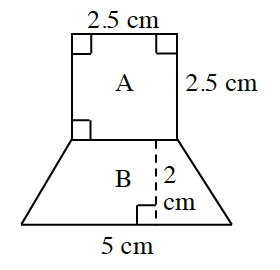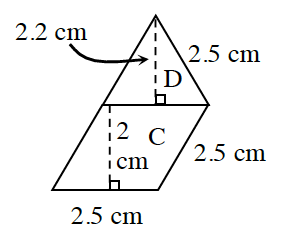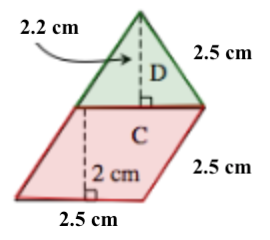### Home > ACC6 > Chapter 5 Unit 12 > Lesson CC2: 5.2.6 > Problem5-84

5-84.

Manuel used pattern blocks to build the shapes below. The block marked A is a square, B is a trapezoid, C is a rhombus (a parallelogram with equal sides), and D is a triangle. Find the area of each of Manuel’s shapes.

1.1.Examine each complex shape in terms of each individual shape within the entire structure and add up the areas to get the total area of Manuel's shapes.

1.Make sure to remember formulas for calculating area:
Square: $\left(\text{base}\right)\left(\text{height}\right)$

Trapezoid: $\frac{1}{2} \text{ height (base a + base b)}$

Triangle: $\frac{1}{2} \text{(base)(height)}$

$13.75$ square cm

Be sure to know why this is the answer from the given measurements.
Now try solving for part (b).

1.